# How exactly machine learns in Machine Learning?

In the previous article (here), We have found answers for the fundamental questions about Machine learning and artificial intelligence, which were :

• What is Machine Learning?
• Why do we need Machine Learning?
• What is the difference between traditional programs and Machine Learning?
• What is the History of Machine Learning, and How it started?
• How Artificial Intelligence and Machine Learning are related?
• What are the applications of Machine Learning?

In this article, we will try to find the answer to another most important question that was not covered in the previous article, which is

## How exactly the machine learns?

Problem Statement: Suppose there are two points in the coordinate plane, (X1, Y1) and (X2, Y2). We want the value of Y for a given X, and (X, Y) should be on the line passing through the given two points.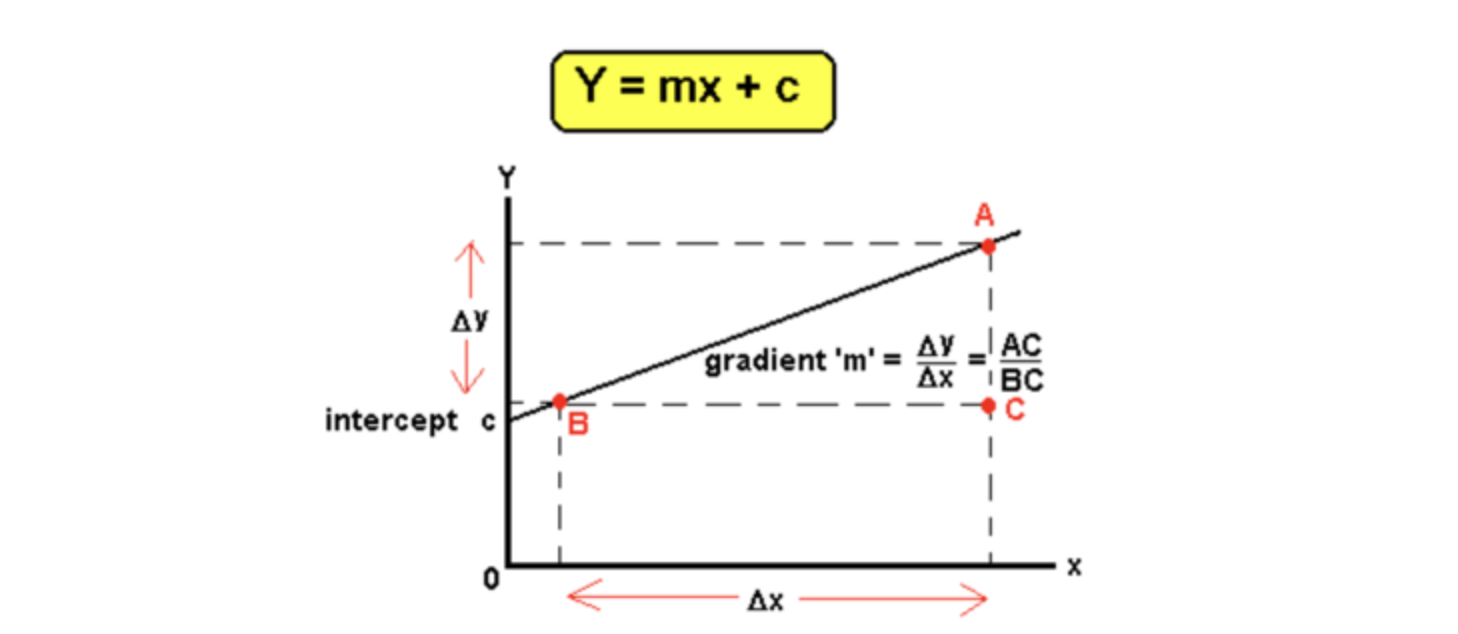Let’s first solve this problem by using the approach that involves traditional programming.

We know Y = m*X + c is the straight-line equation, where m represents slope/gradient, and c is the intercept. One unique line can pass through any given two points (X1, Y1) and (X2, Y2).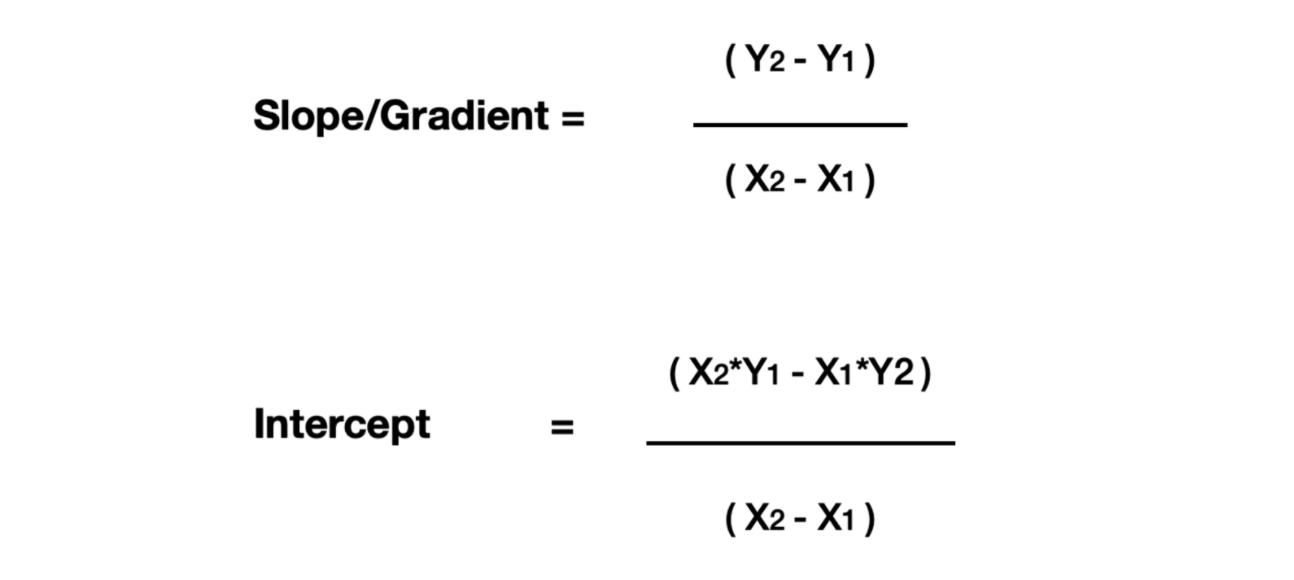We write functions as our programs in the traditional programming approach and based on this function's input, it produces the corresponding output. In the below program, initially, we defined the straight line parameters (slope and gradient), and linear_function is a function that takes the X coordinate as the input and produces the corresponding Y coordinate, which lies on the line Y = m*X + c.

``````# (X1, Y1) Coordinates of first point
# (X2, Y2) Coordinates of second point

slope = (Y2-Y1)/(X2-X1) #X2 != X1
intercept = (X2*Y1 - X1*Y2)/(X2-X1)

def linear_function(X):
Y = slope*X + intercept
return Y
``````

With this approach, traditional programs will find the exact equation of the line. The image below shows a computer where we are writing our functional program and giving it some input. Based on that input, it predicts the output.Now let’s move ahead to know how Machine Learning will solve this problem? Before that, let’s define the term of the regression problem. In regression problems, ML algorithms are expected to learn to predict the continuous output.
While solving the linear regression problem, we can formulate the relationship between any pair of input and output data as Y = W*X + B, where W and B are Weight and Bias matrices. Dimensions of these matrices depend upon the type of problem that we are solving.
With the same analogy, we can also formulate the above problem in the form of Y = W*X + B, where W = Slope/Gradient (m) and B is the intercept ( c ).

In a traditional program, we wrote the value of m and c using the gradient and intercept formula for straight lines, and when we pass the input data into the linear_function function, it produced the output.

For a Machine Learning approach, we must have a set of Input and Output data using which the machine learns a mapping function from input to output set. In simplest words, ML will try to learn the function *linear_function.*

As we know, the requirement of linear_function would be slope m and intercept c. In Machine Learning, the machine will automatically find these variables based on the dataset that we will provide.So, let’s quickly form the dataset using which ML algorithms will try to find m and c,We can easily evaluate that the line's equation, Y = X + 1, fits the above dataset. In other words, we can say m (slope ) = 1 and c (intercept) = 1 is the solution.

Many of you must be thinking that if we already know the output values, why do we need Machine Learning? If we already know the answer, then why do we need a program?

To answer that, let’s go back to our school days. In school textbooks, we usually have examples to learn the theory by analyzing different practice questions. Later, that learning can be tested in the exercise questions or the examination.

For Machine Learning, these initial datasets ( also known as training datasets) in inputs and outputs play the same role as practice examples and their solution. Once the machine learns, it can generate the output for any given value of X as the initial dataset's input.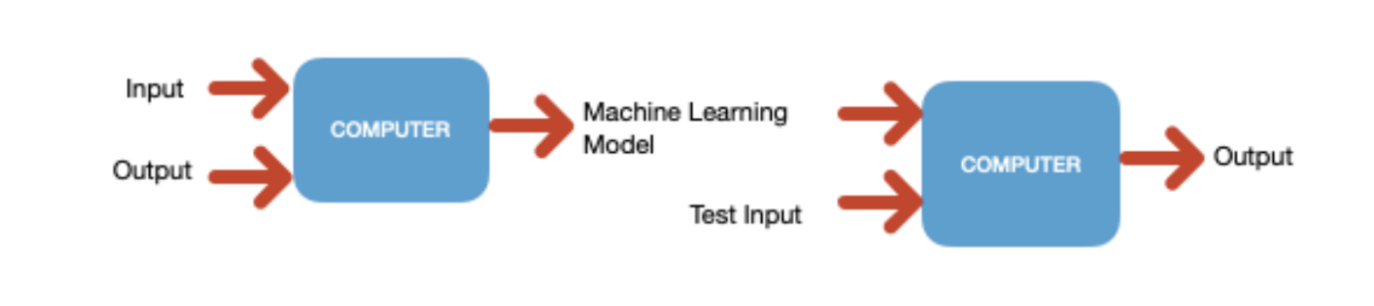Continuing our discussion on how machines will find m and c values?

#### Let’s go through the steps that the machine will follow to find the values of slope and intercept,

Step 1: The machine will choose some random values of m and c ( let’s say, m =0.1 and c = 0.5) and try to find the output Ŷ ( Y_hat) for the given input from the training dataset.

Step 2: It will find the error between the predicted value of Y, which is Ŷ, and the actual/true value of Y, which was present in the training dataset.
This error can be of various types, like Mean Square Error (MSE), Mean Absolute Error (MAE), Root Mean Square Error (RMSE), etc. For a better prediction, this error should be minimum.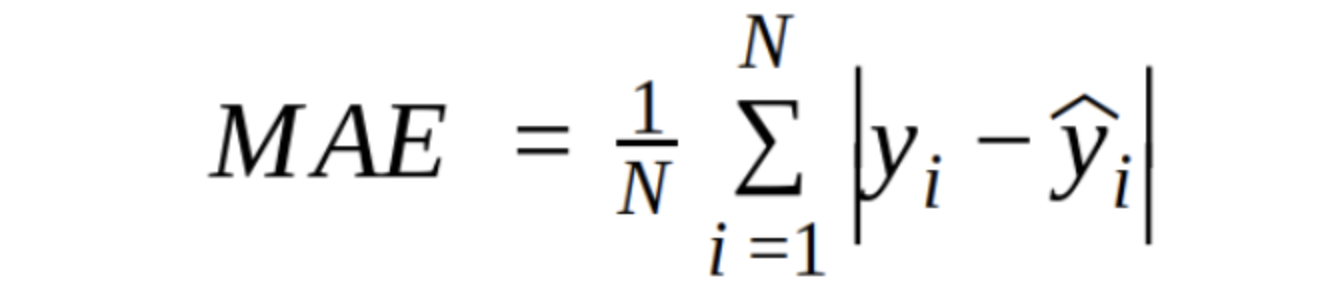Step 3: As we know, there are two parameters based on which MAE varies, i.e., values of slope(m) and the intercept( c ). Consider the above equation as a function of m and c.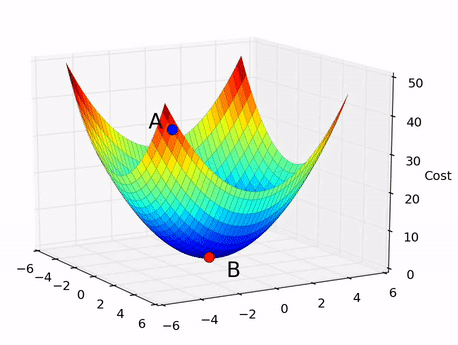We can easily relate the error function (e.g., MAE ) with the cost function as our goal is to minimize it. In the above GIF, m, c and Cost are the three dimensions represented on three axes. Suppose when the machine arbitrarily selected the values for m and c in step 1, we were at position A in the above GIF, and we aim to reach position B, where the cost function is minimum.

Step 4: The machine will update the value of m and c such that the cost function will decrease from the previous value, and then it will again calculate the value of Ŷ on another value of X from the training dataset.

Step 5: The process of updating m and c values and re-calculating the cost function based on the new value of Ŷ will repeatedly iterate until it reaches the point (or nearest to the point) B.

The value of m and c, after reaching a point ( or nearest to the point ) B, will be the learned parameters for the machine. Now, whenever someone gives any finite value of X to our machine learning model, it will predict the value of Y corresponding to every X.

Overall, the machine tried learning the Weight (W) and Bias (B) matrices. This is one special case where both the matrices are constituted of single elements, i.e., m and c.

In the case where W and B will have dimension >1, ML algorithms will try to learn every element that constitutes the weight and bias matrices for that particular data. In the figure below, a11, a12 ….. is the (m X n) matrix elements, and ML will try to learn the value of a11, a12, …., amn.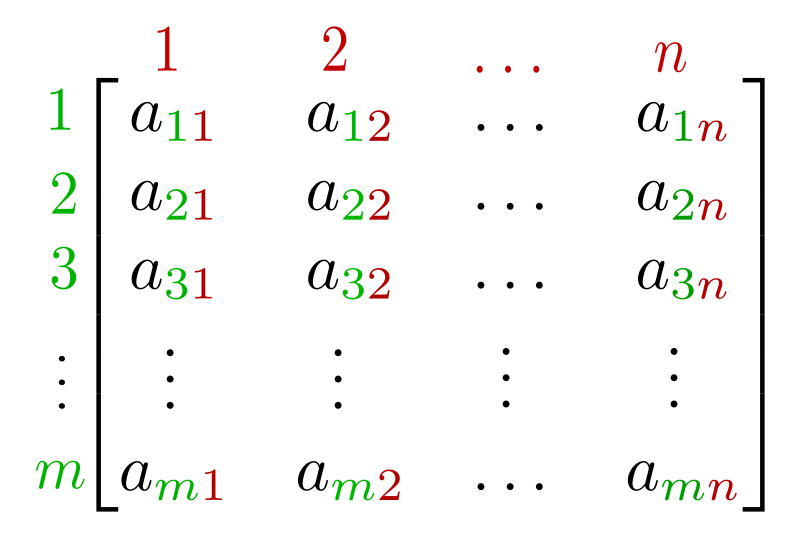And That’s how the machine actually learns, and this ability of learning is termed Machine Learning.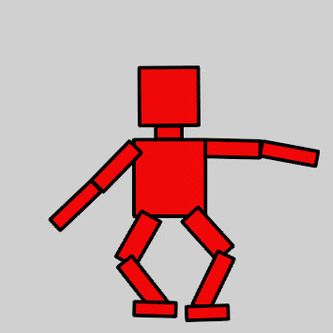Pic Credit: imgur

### Critical questions to explore

Question 1: What are weight and bias matrices?
Question 2: What other options are available in place of MAE?
Question 3: Isn’t it a lengthy process where ML algorithms tweak the value of m and c in every iteration? Can this process be faster? (Hint: Optimisers).
Question 4: What if no one perfect line could fit all the data?

### Conclusion

In this article, we discussed how exactly machine learns in Machine Learning. We solved one common problem of finding the value of straight-line using two different approaches: using traditional programming and the second using ML approach. We also looked at what information Machine will store, which we say as Machine Learning. I hope you have enjoyed the article.

## Enjoy Learning! Enjoy Thinking! Enjoy Algorithms!

Get well-designed application and interview centirc content on ds-algorithms, machine learning, system design and oops. Content will be delivered weekly.